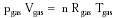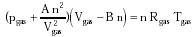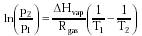Nanomedicine, Volume I: Basic Capabilities

Robert A. Freitas Jr., Nanomedicine, Volume I: Basic Capabilities, Landes Bioscience, Georgetown, TX, 1999

10.3.2 The Van der Waals Equation

The compressibility of gases is most simply described by the well-known ideal gas law:{Eqn. 10.17}

where pgas, Vgas and n are gas pressure, volume, and number of moles, respectively. However, all real gases deviate to some extent from the ideal gas law, even at STP. Most real gases obey the ideal gas law to within a few percent at low densities -- that is, at low pressures (e.g., <~1 atm) and at temperatures well above their condensation points. For real gases at high pressure, finite molecular volumes and intermolecular attractions cause significant deviation from the ideal gas law. In 1873, J.D. van der Waals deduced an empirical equation of state (subsequently derived from statistical mechanics using suitable approximations1031) that reproduces the observed behavior of real gases with moderate accuracy:{Eqn. 10.18}

where A is a measure of intermolecular attraction and B is a measure of finite molecular volumes. The van der Waals gas "constants," given in Table 10.1 for various gases, are known to vary slightly with temperature. Nevertheless, while Eqn. 10.18 is only one of several expressions commonly employed to represent real gas behavior, it is the simplest to use and to interpret. The van der Waals equation remains approximately valid even at temperatures and molar volumes so low that the gas has become a liquid.1031 Note that the critical temperature Tcrit, the highest temperature at which gas and liquid may exist as separate phases at any pressure, is approximated by Tcrit = 8 c1 A / 27 B Rgas, where c1 = 1.01 x 105 J/m3-atm and Rgas = 8.31 J/mole-K; critical pressure pcrit = A / 27 B2.390 For example, in the case of water, at temperatures and pressures above Tcrit = 647.3 K and pcrit = 218.3 atm,763 the vapor and liquid phases become indistinguishable.

The boiling point of a pure liquid as a function of pressure may be approximated by:2036{Eqn. 10.19}

where T1 and T2 are the boiling points (in K) at pressures p1 and p2, respectively, DHvap is the molar heat of vaporization for the liquid (Table 10.8), and Rgas = 8.31 J/mole-K. (This formula assumes that DHvap is constant over the temperature range from T1 to T2.) Taking T1 = 373.16 K, DHvap = 40,690 J/mole, and p1 = 1 atm for water, then at p2 = 6.4 atm the boiling point has risen to T2 = 435 K. Boiling point also is altered by the presence of solute (Section 10.5.3).

Table 10.2 shows the molecular number density achieved inside gas storage vessels maintained at various pressures at Tgas = 310 K. Note that there are significant (near-linear) gains in gas molecule packing density up to 1000 atm, minor (non-linear) gains up to 10,000 atm, and no significant gain at >100,000 atm as density approaches a limiting value for the liquid or solid state. Table 10.2 also shows that pressures calculated using the ideal gas law vary from pressures calculated using the van der Waals equation by ~5% at 100 atm and ~50% at 1000 atm.*

* For comparison, pressures of ~1000 atm occur naturally in the deepest part of the ocean (the Marianas Trench), ~10,000 atm at the crust-mantle interface (the Mohorovic discontinuity), 3.64 million atm at the Earth's core, and ~109 atm at the center of the Sun.

One early experiment provided evidence of stably trapped (Section 10.3.4) room-temperature gases at ~1300 atm pressure inside carbon nanotubes.1169 Single-walled carbon nanotubes have been experimentally charged with trapped hydrogen gas up to 5-10% by weight,2024 and with argon gas at ~600 atm.2034

Last updated on 24 February 2003